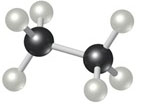# Problem: Calculate the percentage of carbon by mass in each of the compounds represented by the following models. (Carbon atoms are black spheres, hydrogen are grey, oxygen are red.)

🤓 Based on our data, we think this question is relevant for Professor Ingram's class at UWATERLOO.

###### FREE Expert Solution

Mass percent:

Compound is: CH3—H3C or C2H6

• Molar mass C2H6 = 30.07 g/mol###### Problem Details

Calculate the percentage of carbon by mass in each of the compounds represented by the following models. (Carbon atoms are black spheres, hydrogen are grey, oxygen are red.)